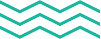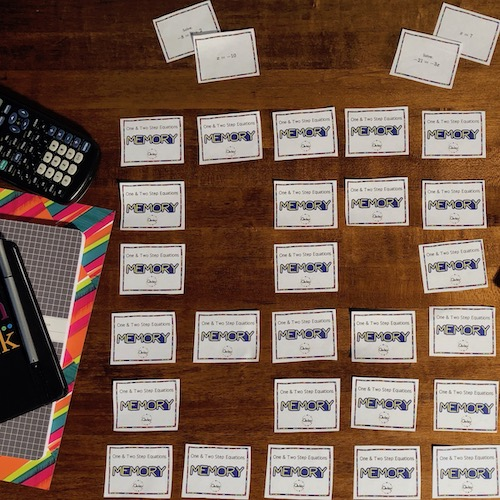•••Activities

### "Flip, Match, and Learn: Make Math Memorable with Qwizy's Math Memory Games!"

• May 15, 2020"Mathematics is not about numbers, equations, computations, or algorithms: it is about understanding." This quote by William Paul Thurston highlights the true essence of teaching mathematics. At Qwizy, we believe in creating materials that go beyond the basic rote memorization of formulas and procedures. Our goal is to help students understand the underlying concepts and develop problem-solving skills that will benefit them beyond the classroom. Our materials, whether it's our Math Memory Games or Holiday Themed Pixel Art Mystery Pictures, are designed to make learning math an enjoyable and engaging experience. So let's ditch the boring routines and make math fun!

Did you know that a simple game of Memory can triple your students' retention abilities? That's right, according to the experts at UT Austin, retrieval practice is the key to helping your students master the content you're presenting them. What is retreval practice? Retrieval practice occurs when students recall something in their mind. Research showes this process has a more powerful effect on students than when they are just rehearsing or reviewing material. So why not make learning more fun with our Math Memory Games?

Our games are structured so that question and answer cards are mixed together in a grid pattern on students' desks. They take turns flipping over cards to match up a question with its corresponding answer. It's a competition to see who can match the most cards and come out on top.

You can play this game individually or in small groups, and each set comes with 15 question cards, 15 answer cards, and a teacher answer sheet for your reference. In my own experience, I've found that this game works wonders for upper-level math classes, especially on tough topics like differentiation of inverse trigonometric functions in AP Calculus.

So get ready to engage your students and watch their retention soar with our Math Memory Games. Let's dive into a few examples of these marvelous retrieval practice activities:

#### Calculus Derivatives: Inverse Trig Math Memory Game

Derivatives Inverse Trig Derivatives Math Memory Game includes all you need to play an exciting game of Memory and review Inverse Trigonometric Derivatives at the same time! Students will be differentiating Inverse Sine, Cosine, and Tangent functions while also applying the Chain Rule.

#### Calculus: Graphs of Derivatives Math Memory Game

Graphs of Derivatives Math Memory Game includes all you need to play an exciting game of Memory and review Functions, Derivatives, and their Graphs at the same time! Students will be analyzing graphs of functions and matching them up the graphs of their first derivatives.

#### Algebra: Graphing Transformations Math Memory Game

Graphing Equations Level 1 Math Review Memory Game includes all you need to play an exciting game of Memory and review Graphing Basic Equations at the same time! Students will be graphing linear and quadratic functions by applying the following transformations: f(x-c), f(x)+d, and -f(x), in addition to graphing exponential functions by changing the values of a and b in equations of the form f(x) = ab^x.

#### Algebra: Slopes From Graphs Math Memory Game

Slopes From Graphs Math Memory Game includes all you need to play an exciting game of Memory and review Slopes of Linear Equations at the same time! Students will be analyzing graphs of linear equations and identifying positive and negative slopes that are both integers and fractions. Additionally, students will need to recognize the equations for vertical and horizontal lines.

#### Multiplying Positive Integers Level 2 Math Memory Game

Multiplying Positive Integers Level 2 Math Memory Game includes all you need to play an exciting game of Memory and review the multiplication of positive integers at the same time! Students will be asked to evaluate the product of expressions containing positive integers ranging from 1 to 50.

For more Math Memory Game activities check out our TPT store. Also, join our newsletter found on Qwizy.com for frequent news and updates. You will receive newsletters with exciting math content and curriculum ideas in addition to informing you about when we have sales or special offers.

References: Schell, Julie A., and Jennifer R. Porter. “Applying the Science of Learning to Classroom Teaching: The Critical Importance of Aligning Learning with Testing.” Journal of Food Science Education, vol. 17, no. 4, 2018, pp. 36–41., doi:10.1111/jfs3.2018.17.issue-4.